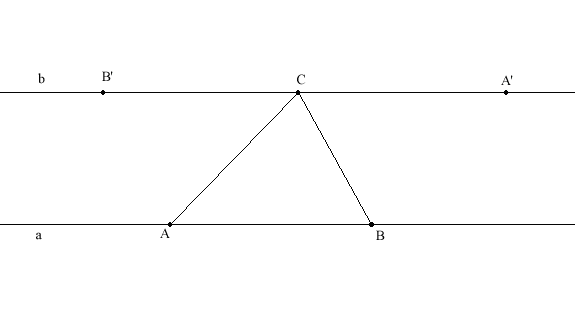# TRIANGLEProof that the sum of the angles in a triangle is 180 degrees

Theorem
If ABC is a triangle then <)ABC + <)BCA + <)CAB = 180 degrees.

Proof
Draw line a through points A and B. Draw line b through point C and parallel to line a.Since lines a and b are parallel, <)BAC = <)B'CA and <)ABC = <)BCA'.
It is obvious that <)B'CA + <)ACB + <)BCA' = 180 degrees.
Thus <)ABC + <)BCA + <)CAB = 180 degrees.

Lemma
If ABCD is a quadrilateral and <)CAB = <)DCA then AB and DC are parallel.

Proof
Assume to the contrary that AB and DC are not parallel.
Draw a line trough A and B and draw a line trough D and C.
These lines are not parallel so they cross at one point. Call this point E.Notice that <)AEC is greater than 0.
Since <)CAB = <)DCA, <)CAE + <)ACE = 180 degrees.
Hence <)AEC + <)CAE + <)ACE is greater than 180 degrees.